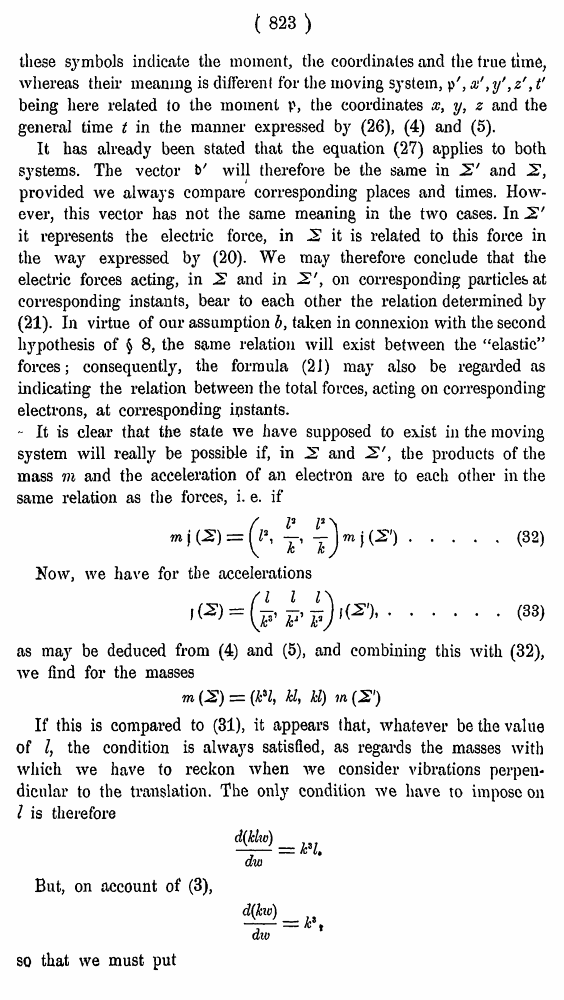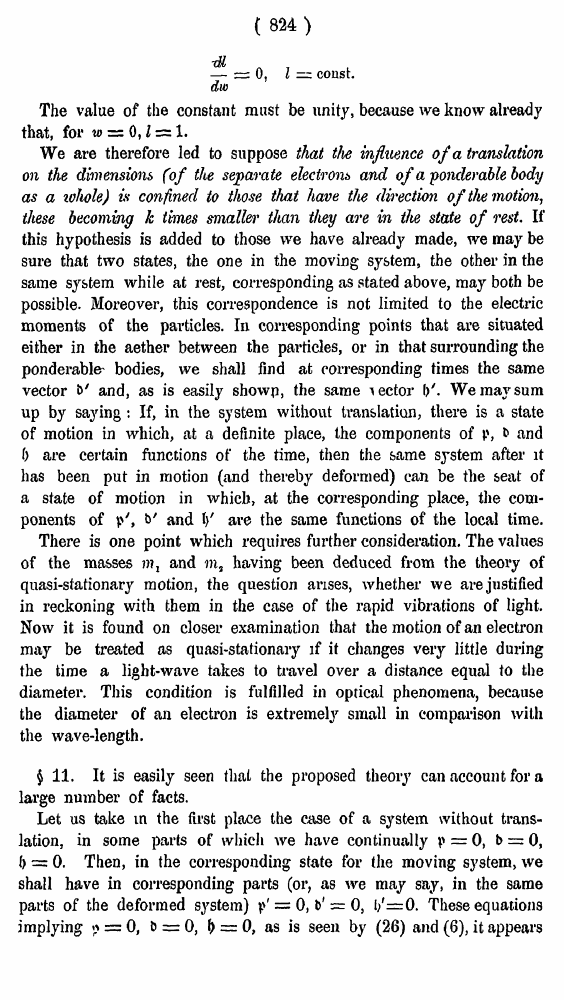# H. A. Lorentz. Electromagnetic phenomena in a system moving with any velocity smaller than that of light. // Proceedings Royal Acad., Amsterdam. Vol. VI., 1904

В начало   Другие форматы   <<<     Страница 823   >>>809  810  811  812  813  814  815  816  817  818  819  820  821  822  823 824  825  826  827  828  829  830  831 ( 823 ) these symbols indicate the moment, the coordinates and the true time, whereas their meaning is different for the moving system, p', x' ,y' ,z' ,t' being here related to the moment p, the coordinates x, y, z and the general time t in the manner expressed by (26), (4) and (5). It has already been stated that the equation (27) applies to both systems. The vector b' will therefore be the same in 2' and 2, provided we always compare corresponding places and times. However, this vector has not the same meaning in the two cases. In 2' it represents the electric force, in 2 it is related to this force in the way expressed by (20). We may therefore conclude that the electric forces acting, in 2 and in 2', on corresponding particles at corresponding instants, bear to each other the relation determined by (21). In virtue of our assumption b, taken in connexion with the second hypothesis of § 8, the same relation will exist between the “elastic” forces; consequently, the formula (21) may also be regarded as indicating the relation between the total forces, acting on corresponding electrons, at corresponding ipstants. - It is clear that the state we have supposed to exist in the moving system will really be possible if, in 2 and 2', the products of the mass Til and the acceleration of an electron are to each other in the same relation as the forces, i. e. if .....(32) Now, we have for the accelerations ......(33) as may be deduced from (4) and (5), and combining this with (32), we find for the masses If this is compared to (31), it appears that, whatever be the value of I, the condition is always satisfied, as regards the masses with which we have to reckon when we consider vibrations perpendicular to the translation. The only condition we have to impose on I is therefore But, on account of (3), so that we must put ( 824 ) The value of the constant must be unity, because we know already that, for w = 0,1 = 1. We are therefore led to suppose that the influence of a translation on the dimensions (of the separate electrons and of a ■ponderable body as a zohole) is confined to those that have the direction of the motion, these becoming k times smaller than they are in the state of rest. If this hypothesis is added to those we have already made, we may be sure that two states, the one in the moving system, the other in the same system while at rest, corresponding as stated above, may both be possible. Moreover, this correspondence is not limited to the electric moments of the particles. In corresponding points that are situated either in the aether between the particles, or in that surrounding the ponderable' bodies, we shall find at corresponding times the same vector &' and, as is easily showp, the same lector I/. We may sum up by saying: If, in the system without translation, there is a state of motion in which, at a definite place, the components of p, b and f) are certain functions of the time, then the same system after it has been put in motion (and thereby deformed) can be the seat of a state of motion in which, at the corresponding place, the components of p', &' and V are the same functions of the local time. There is one point which requires further consideration. The values of the masses m, and m, having been deduced from the theory of quasi-stationary motion, the question arises, whether we are justified in reckoning with them in the case of the rapid vibrations of light. Now it is found on closer examination that the motion of an electron may be treated as quasi-stationary if it changes very little during the time a light-wave takes to travel over a distance equal to the diameter. This condition is fulfilled in optical phenomena, because the diameter of an electron is extremely small in comparison with the wave-length. \$ 11. It is easily seen that the proposed theory can account for a large number of facts. Let us take in the first place the case of a system without translation, in some parts of which we have continually p = 0, t> = 0, () = 0. Then, in the corresponding state for the moving system, we shall have in corresponding parts (or, as we may say, in the same parts of the deformed system) p' = 0, b' = 0, l/=0. These equations implying *> = 0, b = 0, () = 0, as is seen by (26) and (6), it appears